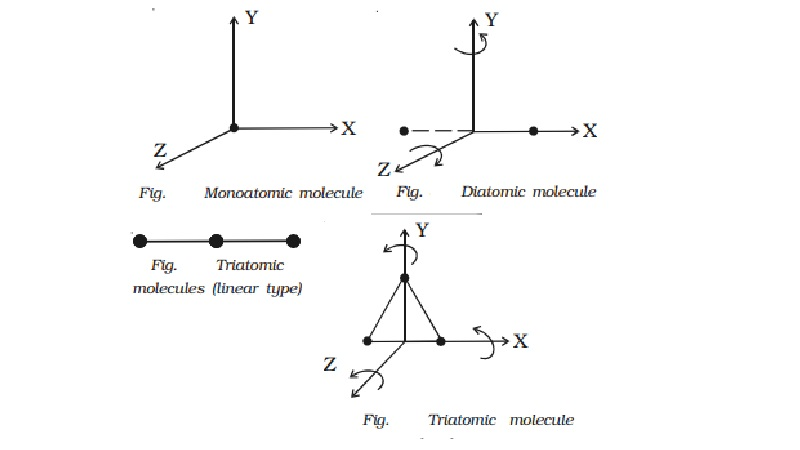Home | | Physics | | Physics | Degrees of freedom of molecule

# Degrees of freedom of moleculeThe number of degrees of freedom of a dynamical system is defined as the total number of co-ordinates or independent variables required to describe the position and configuration of the system.

Degrees of freedom

The number of degrees of freedom of a dynamical system is defined as the total number of co-ordinates or independent variables required to describe the position and configuration of the system.

For translatory motion

(i) A particle moving in a straight line along any one of the axes has one degree of freedom (e.g) Bob of an oscillating simple pendulum.

(ii) A particle moving in a plane (X and Y axes) has two degrees of freedom. (eg) An ant that moves on a floor.

(iii)                A particle moving in space (X, Y and Z axes) has three degrees of freedom. (eg) a bird that flies.

A point mass cannot undergo rotation, but only translatory motion. A rigid body with finite mass has both rotatory and translatory motion. The rotatory motion also can have three co-ordinates in space, like translatory motion ;

Therefore a rigid body will have six degrees of freedom ; three due to translatory motion and three due to rotatory motion.1 Monoatomic molecule

Since a monoatomic molecule consists of only a single atom of point mass it has three degrees of freedom of translatory motion along the three co-ordinate axes as shown in Fig..

Examples : molecules of rare gases like helium, argon, etc.

2 Diatomic molecule

The diatomic molecule can rotate about any axis at right angles to its own axis. Hence it has two degrees of freedom of rotational motion in addition to three degrees of freedom of translational motion along the three axes. So, a diatomic molecule has five degrees of freedom (Fig.). Examples : molecules of O2, N2, CO, Cl 2, etc.

3 Triatomic molecule (Linear type)

In the case of triatomic molecule of linear type, the centre of mass lies at the central atom. It, therefore, behaves like a diamotic molecule with three degrees of freedom of translation and two degrees of freedom of rotation, totally it has five degrees of freedom (Fig.). Examples : molecules of CO2, CS2, etc.

4 Triatomic molecule (Non-linear type)

A triatomic non-linear molecule may rotate, about the three mutually perpendicular axes, as shown in Fig.. Therefore, it possesses three degrees of freedom of rotation in addition to three degrees of freedom of translation along the three co-ordinate axes. Hence it has six degrees of freedom. Examples : molecules of H2O, SO2, etc.

In all the above cases, only the translatory and rotatory motion of the molecules have been considered. The vibratory motion of the molecules has not been taken into consideration.

Study Material, Lecturing Notes, Assignment, Reference, Wiki description explanation, brief detail

Related Topics